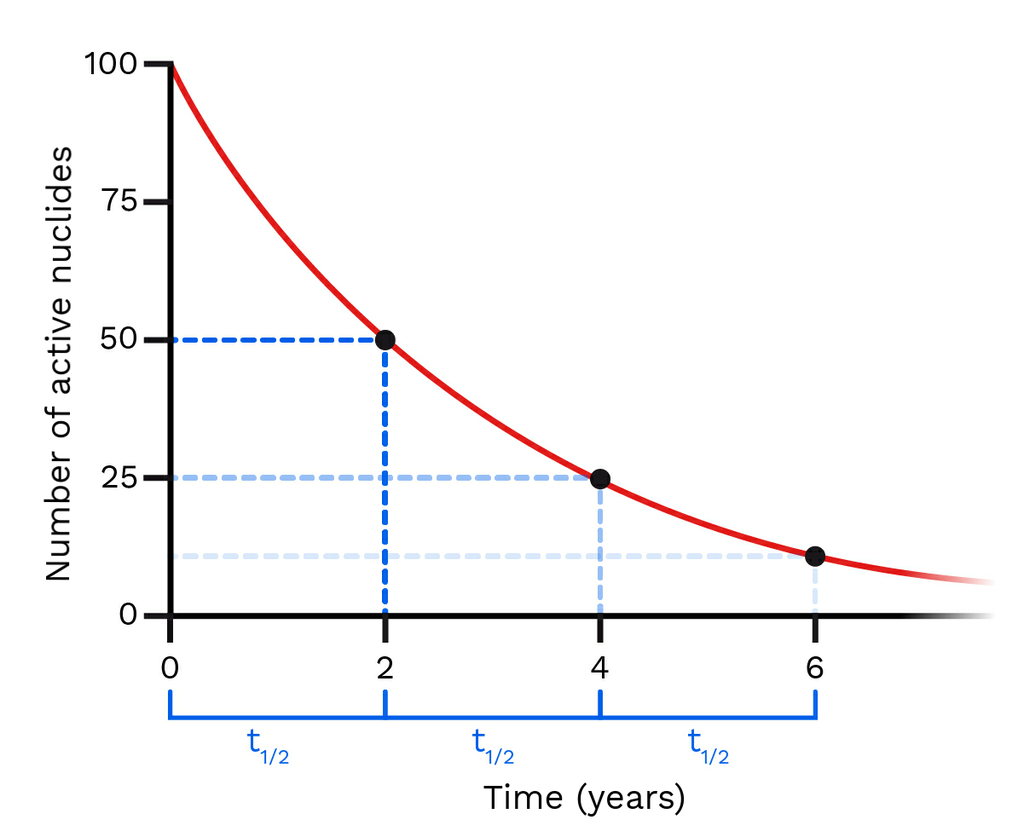The half-life of an active nuclide is the length of time required for half the active nuclides in a sample to decay. Scientists often represent the idea of half-life on a graph. It is a good depiction of how fast a nuclide is decaying and therefore how much alpha, beta, and gamma radiation it is emitting.

On a graph showing the radioactive decay of a nuclide, we can find the half-life of the nuclide by checking the y axis. Remember, after half of the nuclides have decayed, exactly one half-life worth of time has passed. If we draw a line across from the y axis to the curve and then down to the x-axis we can see exactly how long the half-life of our nuclide is!The beauty of the length of time half-life is that no matter where you are on the decay curve, one half-life is ALWAYS the length of time required for exactly half the remaining nuclides to decay.

It is important to recall that as nuclides decay they do not decay into nothingness. They decay into lighter, less energetic daughter nuclides until they reach a stable, strongly bound state.